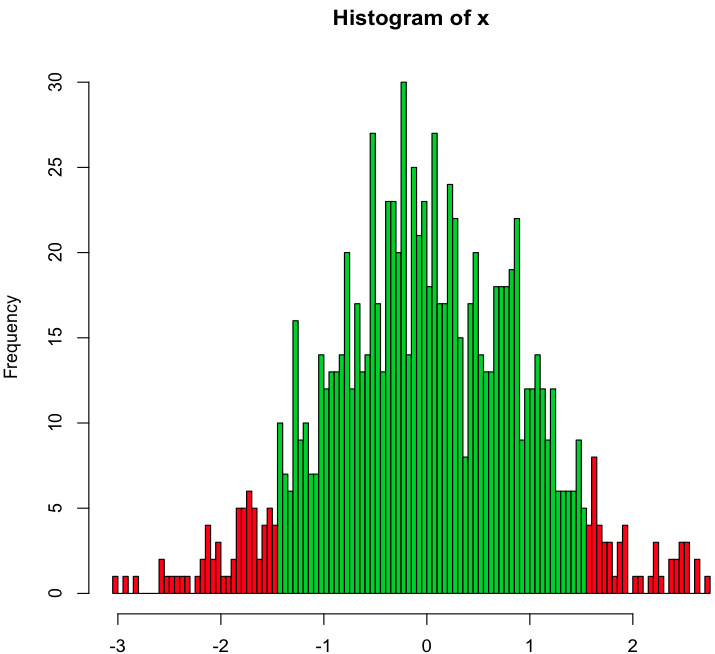# Creating Normal Distribution Using R

In this article we will look at how to create a normal distribution (Histogram) using r programming.

While doing so, we will also review a few important functions of r.

Running the following three commands on the R console will plot the normal distribution.

> x <- rnorm(1000) > h <- hist(x, breaks=100, plot=FALSE) > plot(h, col=ifelse(abs(hx$breaks) < 1.5, 4, 2)) Let’s take a look at each of these commands. Step 1: Generate random numbers > x <- rnorm(1000) In this command we have used the rnorm() function to generate random numbers whose distribution is normal. The argument for the function is the number of random numbers you want to generate, in this case 1000. Apart from specifying the number of random numbers, you can also specify (optional) the mean and standard deviation for the desired distribution. Example: rnorm(4,mean=3,sd=3) Step 2: Create Frequency Table Using the Random Numbers > h <- hist(x, breaks=100, plot=FALSE) For this we will use the histogram function hist(). This function computes a histogram of the given data values. The function can take many arguments. The arguments used by us are x, breaks, and plot. ‘x’ is the vector of values for which the histogram is required. ‘Breaks’ defines the bins for the histogram, and the random numbers are placed in these bins. The breaks argument can be used in a number of ways. For example, we can specify the number of bins we want (breaks=100 in our example). This mostly an approximation. Alternatively you can also specify the exact range and number of each bin. ‘Plot’ defines whether we want the histogram data to be plotted. If the value is false, the graph will not be plotted, only the array data will be stored. In our example, we don’t plot the graph within this function, as we want to perform some more operation on the data while plotting it. So, we just store the data in ‘h’. Step 3: Plot the Distribution, with its tails highlighted in a different color > plot(h, col=ifelse(abs(h$breaks) < 1.5, 4, 2))

Now that we have the data, we can use it to plot it. We can plot any data using the plot function.

‘h’ is the histogram data to be plotted.

‘col’ specifies the color for the histogram bars.

We can specify a single color such as ‘blue’ to plot all bars in blue.

What we want to do here is plot the tails of the histogram in red color. To achieve this, we will supply a vector to the col argument using the if-else statement. h\$breaks specifies the break values. Then we check if this value is less than 1.5. If yes, we color is green (that’s the code 4). If the absolute value is greater than 1.5 we supply the color red (code 2).

Enter the above formula and press enter. The histogram will be plotted as shown below.

You can play around with the formula to see how different variables affect it.

The best part about R is that the graphs are of high quality, and you can simply copy and paste them in your documents. Alternatively, with the base package, you can save them as a PDF.In this example, we just used random data to plot the distribution. In reality, we can supply our own data to plot the graphs.